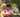### JavaScript array map & filter methods

December 04, 2019

JavaScript-Map-Method

The map() method creates a new array with the results of calling a provided function on every element in the calling array.

A classic example is if we want an array of the square of all elements in a given array, we could do this using a for-loop as seen in the example below:

``````    const array = [2, 5, 9];
let squares = [];
for (let i = 0; i < array.length; i++) {
squares.push(array[i] * array[i]));
}
console.log(squares); // [4, 25, 81]
console.log(array); // [2, 5, 9]``````

What does the above achieve? It loops through an array, finds the square of each element in the array and pushes it to the squares array that was earlier defined.

This is similar to what the map function achieves only that you do not need to define another array that the result is pushed to. The map function automatically does that. So let us accomplish the above using the map function.

``````    const array = [2, 5, 9];
let squares = array.map((num) => num * num);
console.log(squares); // [4, 25, 81]
console.log(array); // [2, 5, 9]``````

Given that there are other similar array methods like the ForEach method, you might wonder, "when should I use (or not) the map method?" Here are some questions that can help you decide:

• Do I need an array to be returned from the method and will the returned array be used?
• Am I returning a value from the callback function?

If your answer to any of these questions is Yes, you should use the map function. If your answer is negative in both cases, you should probably use forEach or for..loop instead.

Example 1: Extracting values from an array of objects

We want to extract certain values from an array of objects. For instance, in a array of girls, we want to get the ages of the girls.

``````    const girls = [
{name: 'Sarah', age: 19},
{name: 'Laura', age: 10},
{name: 'Jessy', age: 29},
{name: 'Amy', age: 23}];

let girlsAges = girls.map((girl) => girl.age);``````

Example 2: Apply the Callback on only certain elements

If we want to the callback to only be applied to certain elements in an array, say odd numbers, we can use an if statement to do this.

``````    const numbers = [4, 9, 36, 49];

let oddSquareRoots = numbers.map((num) => {
if(num % 2 != 0) {
return Math.sqrt(num)
}
return num;
})

console.log(oddSquareRoots);``````

or using ternary operators

``````    const numbers = [4, 9, 36, 49];

let oddSquareRoots = numbers.map((num) => {
return num % 2 !== 0 ? Math.sqrt(num) : num
})

console.log(oddSquareRoots);``````

However, a more efficient way to achieve this is using the JavaScript Array Filter method.

JavaScript-Filter-Method

The filter() method creates a new array with all elements that pass the test implemented by the provided function.

If you have an array of random numbers (e.g ages of people) and you only need numbers that are above a certain number ( e.g 18). How will you do this using the normal for-loop?

``````    const ages = [11, 34, 8, 9, 23, 51, 17, 40, 14];
let olderThan18 = [];
for (let i = 0; i < ages.length; i++) {
if(ages[i] > 18){
olderThan18.push(ages[i])
}
}``````

This is much simpler using the filter method as shown below:

``````    const ages = [11, 34, 8, 9, 23, 51, 17, 40, 14];
let olderThan18 = ages.filter((age) => age > 18);
console.log(olderThan18); //[34, 23, 51, 40]``````

If we decide to declare the callback function before using it with the filter method, we can do this:

``````    const ages = [11, 34, 8, 9, 23, 51, 17, 40, 14];
const isOlderThan18 = (age) => age > 18;
let olderThan18 = ages.filter(isOlderThan18);
console.log(olderThan18); // [34, 23, 51, 40]``````

Example 1: Filtering an array of objects

If you have an array of countries and you want only countries that are in a particular continent. This is an example of how you can do that using array filter method.

``````    const countries = [
{ name: 'Nigeria', continent: 'Africa'},
{ name: 'Nepal', continent: 'Asia'},
{ name: 'Angola', continent: 'Africa'},
{ name: 'Greece', continent: 'Europe'},
{ name: 'Kenya', continent: 'Africa'},
{ name: 'Greece', continent: 'Europe'}
]
let asianCountries = countries.filter(country => {
return country.continent === 'Asia';
})
console.log(asianCountries); // [{name: "Nepal", continent: "Asia"}]``````

Example 2: Search for specific letters in an array

Given an array of words and you want to find out which of the words contains specific letters. For example, you want female names that contain specific alphabets together, here is how you can do it.

``````    const names = ['Victoria', 'Pearl', 'Olivia', 'Annabel', 'Sandra', 'Sarah'];
const filterItems = (letters) => {
return names.filter(name => name.indexOf(letters) > -1);
}
console.log(filterItems('ia')); // ["Victoria", "Olivia"]``````Posted by Tai Lu ( 呂台生 ) who's building useful things.You should follow him on Twitter